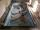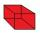# Volume + surface area - math problems

#### Number of problems found: 260

• Pool coatingHow many tiles 25 cm × 15 cm need to coat the bottom and sidewalls of the pool with bottom dimensions 30 m × 5 m, if the pool can fit up to 271500 liters of water?
• Center of the cubeThe Center of the cube has a distance 16 cm from each vertex. Calculate the volume V and surface area S of the cube.
• BallsThree metal balls with volumes V1=71 cm3 V2=78 cm3 and V3=64 cm3 melted into one ball. Determine it's surface area.
• Rotary coneThe volume of the rotation of the cone is 472 cm3, and the angle between the side of the cone and the base angle is 70°. Calculate the lateral surface area of this cone.
• ConeCalculate volume and surface area of ​​the cone with a diameter of the base d=15 cm and side of the cone with the base has angle 52°.
• TerezaThe cube has an area of base 256 mm2. Calculate the edge length, volume, and area of its surface.
• PrismRight-angled prism, whose base is a right triangle with leg a = 3 cm and hypotenuse c = 13 cm, has the same volume as a cube with an edge length of 3 dm. a) Find the height of the prism b) Calculate the surface of the prism c) What percentage of the cube'
• Cube zoomHow many percents do we increase the volume and surface of the cube if we magnify its edge by 38 %?
• Rectangular cuboidThe rectangular cuboid has a surface area 5334 cm2, and its dimensions are in the ratio 2:4:5. Find the volume of this rectangular cuboid.
• CubesOne cube is an inscribed sphere and the other one described. Calculate the difference of volumes of cubes, if the difference of surfaces in 257 mm2.
• Axial sectionThe axial section of the cone is an equilateral triangle with area 168 cm2. Calculate the volume of the cone.
• Cone A2VThe surface of the cone in the plane is a circular arc with central angle of 126° and area 415 cm2. Calculate the volume of a cone.
• RotationThe right triangle with legs 11 cm and 18 cm rotate around the longer leg. Calculate the volume and surface area of the formed cone.
• SphereThe surface of the sphere is 12100 cm2, and the weight is 136 kg. What is its density?
• Special cubeCalculate the edge of cube, if its surface and its volume is numerically equal number.
• CubeThe sum of lengths of cube edges is 57 cm. What is its surface and volume?
• Sphere slicesCalculate the volume and surface of a sphere if the radii of parallel cut r1=31 cm, r2=92 cm, and its distance v=25 cm.
• Cylinder - A&VThe cylinder has a volume 1287. The base has a radius 10. What is the area of the surface of the cylinder?
• Cylinder - basicsCylinder with base radius r = 54 cm and height h=35 cm. Calculate: a) Area of the base
• Sphere A2VThe surface of the sphere is 241 mm2. What is its volume?

Do you have an exciting math question or word problem that you can't solve? Ask a question or post a math problem, and we can try to solve it.

We will send a solution to your e-mail address. Solved examples are also published here. Please enter the e-mail correctly and check whether you don't have a full mailbox.

Tip: Our volume units converter will help you with the conversion of volume units. Volume - math word problems. Examples for the calculation of the surface area of ​​the solid object .